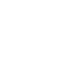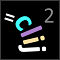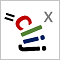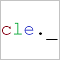# CLIJ2GPU accelerated image processing for everyone

CLIJ2 home

## generateProximalNeighborsMatrixProduces a touch-matrix where the neighbors within a given distance range are marked as touching neighbors.

Takes a distance matrix (e.g. derived from a pointlist of centroids) and marks for every column the neighbors whose distance lie within a given distance range (>= min and <= max). The resulting matrix can be use as if it was a touch-matrix (a.k.a. adjacency graph matrix).

### Parameters

distance_marix : Image touch_matrix_destination : Image min_distance : float, optional default : 0 max_distance : float, optional default: 10

## Returns

touch_matrix_destination

Categories: Graphs, Measurements

Availability: Available in Fiji by activating the update sites clij and clij2. This function is part of clij2_-2.5.0.1.jar.

### Usage in ImageJ macro

``````Ext.CLIJ2_generateProximalNeighborsMatrix(Image distance_matrix, Image touch_matrix_destination, Number min_distance, Number max_distance);
``````

### Usage in object oriented programming languages

Java
```// init CLIJ and GPU
import net.haesleinhuepf.clij2.CLIJ2;
import net.haesleinhuepf.clij.clearcl.ClearCLBuffer;
CLIJ2 clij2 = CLIJ2.getInstance();

// get input parameters
ClearCLBuffer distance_matrix = clij2.push(distance_matrixImagePlus);
touch_matrix_destination = clij2.create(distance_matrix);
float min_distance = 1.0;
float max_distance = 2.0;
```
```// Execute operation on GPU
clij2.generateProximalNeighborsMatrix(distance_matrix, touch_matrix_destination, min_distance, max_distance);
```
```// show result
touch_matrix_destinationImagePlus = clij2.pull(touch_matrix_destination);
touch_matrix_destinationImagePlus.show();

// cleanup memory on GPU
clij2.release(distance_matrix);
clij2.release(touch_matrix_destination);
```
Matlab
```% init CLIJ and GPU
clij2 = init_clatlab();

% get input parameters
distance_matrix = clij2.pushMat(distance_matrix_matrix);
touch_matrix_destination = clij2.create(distance_matrix);
min_distance = 1.0;
max_distance = 2.0;
```
```% Execute operation on GPU
clij2.generateProximalNeighborsMatrix(distance_matrix, touch_matrix_destination, min_distance, max_distance);
```
```% show result
touch_matrix_destination = clij2.pullMat(touch_matrix_destination)

% cleanup memory on GPU
clij2.release(distance_matrix);
clij2.release(touch_matrix_destination);
```
Icy JavaScript
```// init CLIJ and GPU
importClass(net.haesleinhuepf.clicy.CLICY);
importClass(Packages.icy.main.Icy);

clij2 = CLICY.getInstance();

// get input parameters
distance_matrix_sequence = getSequence();
distance_matrix = clij2.pushSequence(distance_matrix_sequence);
touch_matrix_destination = clij2.create(distance_matrix);
min_distance = 1.0;
max_distance = 2.0;
```
```// Execute operation on GPU
clij2.generateProximalNeighborsMatrix(distance_matrix, touch_matrix_destination, min_distance, max_distance);
```
```// show result
touch_matrix_destination_sequence = clij2.pullSequence(touch_matrix_destination)
```import pyclesperanto_prototype as cle